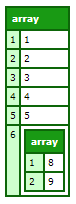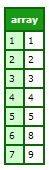#### Description

Appends an array element to an array.  Concatenates arrays when the merge argument is set to true and the value argument is an array.

#### Returns

True, on successful completion.

#### Function syntax

ArrayAppend(array, value [,merge])

ArrayPrependAdding elements to an array in Basic array techniques in the Developing ColdFusion Applications

#### History

Coldfusion 10: Added the merge argument

ColdFusion MX: Changed behavior: this function can be used on XML objects.

#### Parameters

Parameter

Description

array

Name of an array

value

Value to add at end of array

merge

If set to true, and value parameter is an array, appends array elements individually to the source array. If false (default) the complete array is added as one element at the end, in the source array. If value is not an array this argument is ignored.

#### Example 1

```<cfscript>
myArray=[1,2,3,4,5];
ArrayAppend(myArray,6,"true");
writeoutput(serializeJSON(myArray)); //adds the value 6 to myArray
</cfscript>```

#### Output

[1,2,3,4,5,6]

```<cfscript>
myArray=[1,2,3,4,5];
ArrayAppend(myArray,[8,9],"false"); // merge=false
writedump(myArray) //adds the new array as a single element
</cfscript>```

Output```<cfscript>
myArray=[1,2,3,4,5];
ArrayAppend(myArray,[8,9],"true"); // merge=true
writedump(myArray) //adds the new array elements as individual elements
</cfscript>```

Output